Chapter 2

We no longer regard the electron as a point-like particle. The electron has an internal structure, and the electric charge inside the electron has continuum distribution.

Here is one of our most basic assumptions about the electron; the electric charge distribution inside of the electron has the following equation:We use the spherical polar coordinates in our book, in which r is the radial coordinate,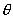is the polar angle, e is the electric charge of the electron,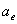is the electron radius constant.

By integrating the electric charge density equation (2.1), we can find the volume electric charge: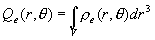(2.2)

In which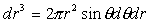, thus: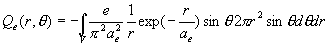(2.3)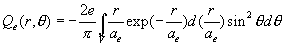(2.4)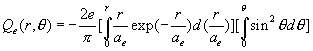(2.5)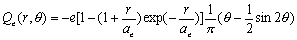(2.6)

The equation (2.6) is the electric charge space distribution equation.
From equation (2.6), we find out that:

When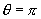, therefore: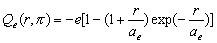(2.7)

As we can see, the electric charge distribution is a kind of cumulative gamma distribution [B]

Equation (2.7) is the electric charge equation within the sphere of radius r.

When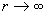, thus:(2.8)

Thus we can see that the electron as a whole has one unit negative electric charge e.